# Excel教程案例：每月流水统计（出货数、不良率、检查天数等）

[日期：2015-12-25]   来源：IT部落窝  作者：IT部落窝   阅读：1259[字体： ]

excel统计每月数量情况在工作中非常常见。通常来说，月度统计表一般是交给上级领导，而年度统计表是要交给老板，性质就不同了。在统计的时候，卢子都是尽可能全面统计，让老板可以随时查看到所需要的数据。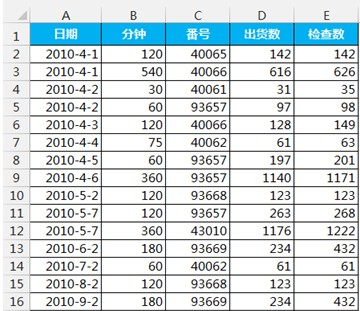excel每月流水统计案例：根据明细表统计2011年每个月份的检查天数、检查时间（h）、出货数、检查数、不良数、不良率。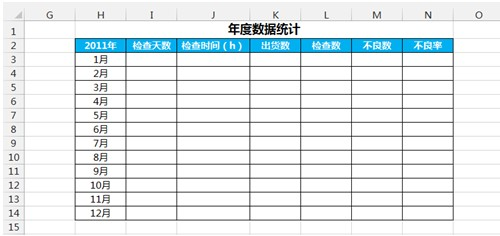=COUNTIF(A\$2:A2,A2)=1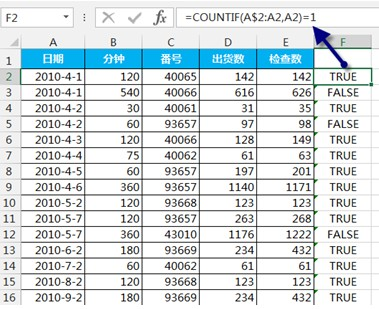=SUMPRODUCT((TEXT(\$A\$2:\$A\$35,"e年m月")=\$H\$2&H3)*\$F\$2:\$F\$35)

=ROUND(SUMPRODUCT((TEXT(\$A\$2:\$A\$35,"e年m月")=\$H\$2&H3)*\$B\$2:\$B\$35)/60,0)

=SUMPRODUCT((TEXT(\$A\$2:\$A\$35,"e年m月")=\$H\$2&H3)*\$D\$2:\$D\$35)

=SUMPRODUCT((TEXT(\$A\$2:\$A\$35,"e年m月")=\$H\$2&H3)*\$E\$2:\$E\$35)

=L3-K3

=IF(L3=0,"",TEXT(M3/L3,"0.0%"))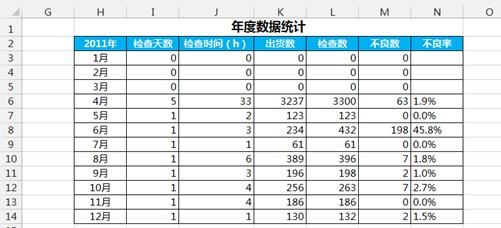COUNTIF函数语法：
=COUNTIF(去哪里数，按什么条件数)

=COUNTIF(C:C,40065)
ROUND函数语法：
=ROUND(表达式或单元格,N)
N就是精确到小数点N位，并四舍五入。以0为分界点，0就是没有小数点，2就是小数点2位，-2就是十位数的四舍五入。负数比较少用，举个例子来说明。
=ROUND(123.45,-2)
-2表示十位数四舍五入，十位数为2，小于5，所以舍去，结果为100。
=ROUND(153.45,-2)

IF函数语法：
=IF(条件判断，如果条件成立的值，反之（即条件不成立）的值)
IF函数是一个使用频率非常高的函数，经常借助这个函数进行判断。如成绩大于80的显示优秀，其他显示一般。
=IF(A1>80,"优秀","一般")

Q:SUMPRODUCT函数这么牛，各种条件求和都能搞定，那它可以进行条件计数吗？
A:答案是肯定的，SUMPRODUCT函数是一个非常好用的函数，求和计数都可以。称他为条件求和、计数之王也不过分。

=SUMPRODUCT((条件1)*(条件2)* (条件3)*……*(条件N))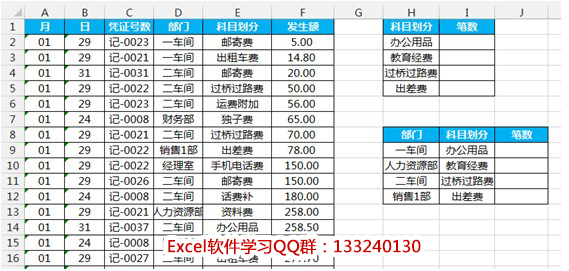1、根据明细表统计科目划分的笔数，在I2输入公式，双击填充公式。
=SUMPRODUCT(--(\$E\$2:\$E\$53=H2))
2、根据明细表统计各部门科目划分的笔数，在J9输入公式，双击填充公式。
=SUMPRODUCT((\$D\$2:\$D\$53=H9)*(\$E\$2:\$E\$53=I9))

该篇教程的excel统计每月数量案例是非常实用的行业案例，分享给大家学习。更多的教程也可以关注下方的微信公众号：获取。分享到： QQ空间 新浪微博 腾讯微博 人人网